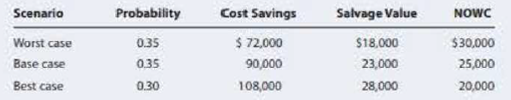Chapter 12, Problem 19PFundamentals of Financial Manageme...

14th Edition
Eugene F. Brigham + 1 other
ISBN: 9781285867977

Solutions

Chapter
SectionFundamentals of Financial Manageme...

14th Edition
Eugene F. Brigham + 1 other
ISBN: 9781285867977
Textbook Problem

NEW PROJECT ANALYSIS Holmes Manufacturing is considering a new machine that costs $250,000 and would reduce pretax manufacturing costs by$90,000 annually. Holmes would use the 3-year MACRS method to depreciate the machine, and management thinks the machine would have a value of $23,000 at the end of its 5-year operating life. The applicable depreciation rates are 33%, 45%, 15%, and 7%, as discussed in Appendix 12A. Net operating working capital would increase by$25,000 initially, but it would be recovered at the end of the project's 5-year life. Holmes's marginal tax rate is 40%, and a 10% WACC is appropriate for the project. a. Calculate the project's NPV, IRR. M1RR, and payback. b. Assume management is unsure about the $90,000 cost savings—this figure could deviate by as much as plus or minus 20%. What would the NPV be under each of these situations? c. Suppose the CFO wants you to do a scenario analysis with different values for the cost savings, the machine's salvage value, and the net operating working capital (NOWC) requirement. She asks you to use the following probabilities and values in the scenario analysis:Calculate the project’s expected NPV, its standard deviation, and its coefficient of variation. Would you recommend that the project be accepted? Why or why not? a. Summary Introduction To Determine: The project’s NPV, IRR, MIRR and Payback Period. Introduction: The net present value is also termed as the discounted cash flow approach is a mainstream capital budget method that considers the time value of cash. It utilizes net present value of the investment as the base to accept or reject a projected investment in projects like buying of new machine, buying of stock or inventory etc. Explanation Determine the cash flows of the project from year 0 to year 5 Using a excel spreadsheet, the cash flow for year 0 is determined as -$275,000, year 1 is determined as $87,000, year 2 is determined as$99,000, year 3 is determined as $69,000, year 4 is determined as$61,000, year 5 is determined as $92,800. Excel Spreadsheet: Excel Workings: Therefore the cash flow for year 0 is -$275,000, year 1 is $87,000, year 2 is$99,000, year 3 is $69,000, year 4 is$61,000, year 5 is \$92,800...

b.

Summary Introduction

To Determine: The NPV if the savings increase by 20% and the NPV if the savings decrease by 20%.

c.

Summary Introduction

To Determine: The expected NPV, standard deviation and coefficient of variation.

Still sussing out bartleby?

Check out a sample textbook solution.

See a sample solution

The Solution to Your Study Problems

Bartleby provides explanations to thousands of textbook problems written by our experts, many with advanced degrees!

Get Started

What does the invisible hand of the marketplace do?

Brief Principles of Macroeconomics (MindTap Course List)

Why must researchers document their sources meticuousIy?

Essentials of Business Communication (MindTap Course List)

Define Web Services.

Accounting Information Systems

YIELD TO CALL Six years ago the Singleton Company issued 20-year bonds with a 14% annual coupon rate at their 1...

Fundamentals of Financial Management, Concise Edition (with Thomson ONE - Business School Edition, 1 term (6 months) Printed Access Card) (MindTap Course List)

What is activity-based product costing?

Cornerstones of Cost Management (Cornerstones Series)